### 4.4Reprogrammable Logic Using STT-MTJs

Recently, it has been demonstrated that by using direct communication between STT-MTJs, logic operations can be realized for which the MTJ devices are used simultaneously as memory and computing elements in intrinsic logic-in-memory architectures. In  and  experimental demonstrations of two-input and three-input reprogrammable logic gates (Fig. 4.18) are reported to realize the basic Boolean logic operations AND, OR, NAND, NOR, and the Majority operation. This section describes the operating principle and presents a reliability analysis of the reprogrammable gates.

The basic Boolean logic operations using reprogrammable gates are executed in two sequential steps. These steps comprise an appropriate preset operation (parallel or antiparallel state) in the output MTJ and then applying a voltage pulse () with a proper amplitude to the gate. Depending on the logic states of the input MTJs (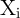), the preset in the output MTJ (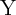), and the voltage level applied to the gate, a conditional switching behavior in the output MTJ is provided that corresponds to a particular logic operation .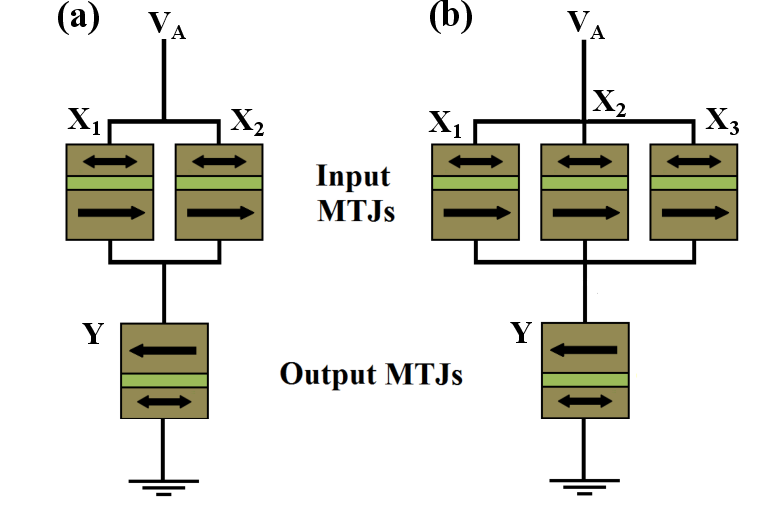Figure 4.18.: STT-MTJ-based (a) two-input and (b) three-input reprogrammable logic gates. X(Y) shows an input (output) MTJ.

 Table 4.1.: Realized conditional switching behavior is equivalent to the AND and OR operations with a preset of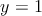using the two-input reprogrammable gate. Desired switching events in the output () are indicated by boldface type.

 Input Patterns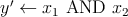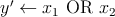Step 1 Step 2 Step 1 Step 2 State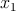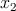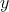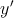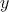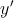1 LRS (0) LRS (0) HRS (1) LRS (0) HRS (1) LRS (0) 2 LRS (0) HRS (1) HRS (1) LRS (0) HRS (1) HRS (1) 3 HRS (1) LRS (0) HRS (1) LRS (0) HRS (1) HRS (1) 4 HRS (1) HRS (1) HRS (1) HRS (1) HRS (1) HRS (1)

Table 4.1 and Table 4.2 illustrate how the AND, OR, NAND, and NOR operations are performed employing the two-input reprogrammable gate in two steps. The variable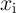show the logic states of an input MTJ (X) and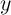represents the logic state of the output MTJ (Y). In order to perform a logic operation, first a preset of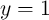(setting Y in the high-resistance state (HRS) shown in Table 4.1) or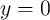(setting Y in the low-resistance state (LRS) shown in Table 4.2) is performed in the output MTJ (Step 1). In Step 2 a proper voltage level (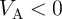or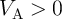with optimized amplitude explained in Section 4.3.2) is applied to the gate to enforce the desired (high-to-low or low-to-high) resistance switching event in the output MTJ to execute the logic operation AND/OR or NAND/NOR. Compared to the (N)AND operation, the (N)OR operation requires a lower voltage amplitude (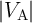), as it must enforce a desired switching event only, if both input MTJs are in the low resistance state (State 1). For the (N)AND operation the switching events are enforced not only in State 1 but also when only one of the inputs is in the low resistance state (State 2 and State 3). These switching events in State 2 and State 3 are desired switching events for the (N)AND operation, while they are undesired events for the (N)OR operation.

 Table 4.2.: Realized conditional switching behavior is equivalent to the NAND and NOR operations with a preset of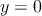using the two-input reprogrammable gate.

 Input Patterns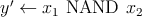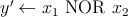Step 1 Step 2 Step 1 Step 2 State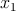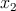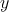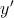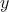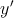1 LRS (0) LRS (0) LRS (0) HRS (1) LRS (0) HRS (1) 2 LRS (0) HRS (1) LRS (0) HRS (1) LRS (0) LRS (0) 3 HRS (1) LRS (0) LRS (0) HRS (1) LRS (0) LRS (0) 4 HRS (1) HRS (1) LRS (0) LRS (0) LRS (0) LRS (0)

Conditional switching corresponding to specific logic operations in reprogrammable logic gates (Fig. 4.18) are performed by applying a proper voltage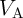to the gates. For given MTJ device characteristics, the value of the circuit parameter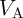has to be optimized to ensure a reliable conditional switching behavior of the output MTJ for any possible input pattern. Indeed, for any logic operation performed by the reprogrammable gates, this optimization is required to maximize (minimize) the switching probability in the output MTJ (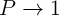or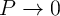), when it is a desired (an undesired) switching event in Step 2 (Table 4.1 and Table 4.2). Therefore, for the reliability analysis, the error probability of a given input state (State) is defined as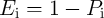(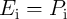) for a desired (undesired) switching event, where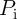is the switching probability of the output MTJ in State. It should be noted that the input MTJs are left unchanged and thus their switching probabilities are negligible as the current flowing through the output MTJ splits between the inputs, and their currents are below the critical current required for the STT switching.

Similar to the implication gates (Eq. 4.21), by assuming equal incidence probabilities for all input patterns, we obtain the average error probability (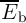) of a basic logic operation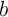implemented by the reprogrammable gate as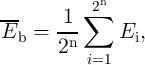(4.22)

where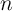is the number of input MTJs. By using Table 4.1, Table 4.2, and Eq. 4.22,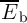is obtained for the two-input (N)OR and (N)AND operations as

 EOR = ENOR =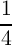∑ i=14E i =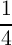[(1 - P1) + P2 + P3 + P4], (4.23) EAND = ENAND =∑ i=14E i =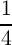[(1 - P1) + (1 - P2) + (1 - P3) + P4], (4.24)
where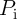is the switching probability of the output MTJ in State.

It is important to note that for the AND and OR operations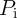represents the probability for antiparallel to parallel (AP–to–P) magnetization switching, while it is the probability for the P–to–AP switching in the case of NAND and NOR operations. In order to calculate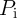for different input patterns and various logic operations, we use Eq. 4.15 which express the MTJ’s switching probability as a function of the applied current. For calculating the current flowing through each MTJ, the voltage-dependent effective TMR model (Eq. 4.18) is used coupled with Kirchhoff’s laws applied to the two- and three-input reprogrammable gates (Fig. 4.18).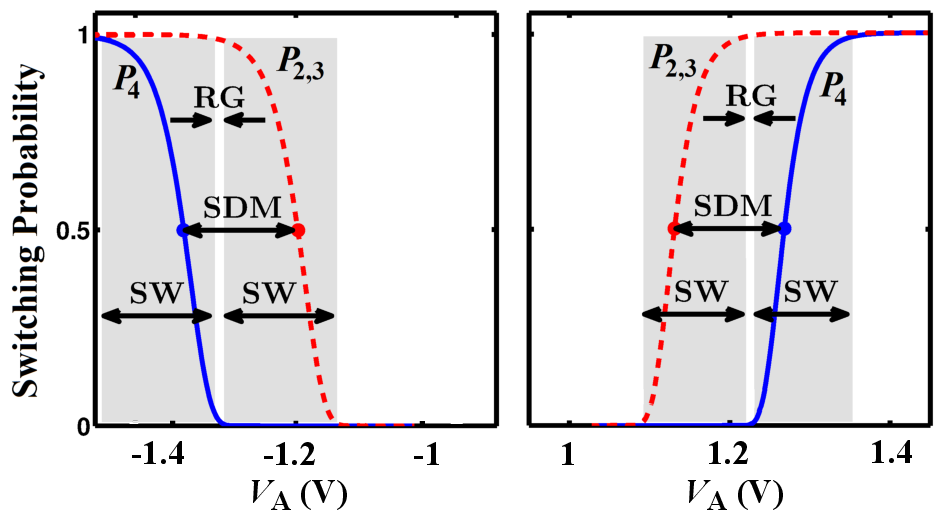Figure 4.19.: Switching probabilities of the nearest desired (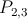) and undesired (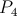) switching events shown for the AND (left side) and NAND (right side) operations.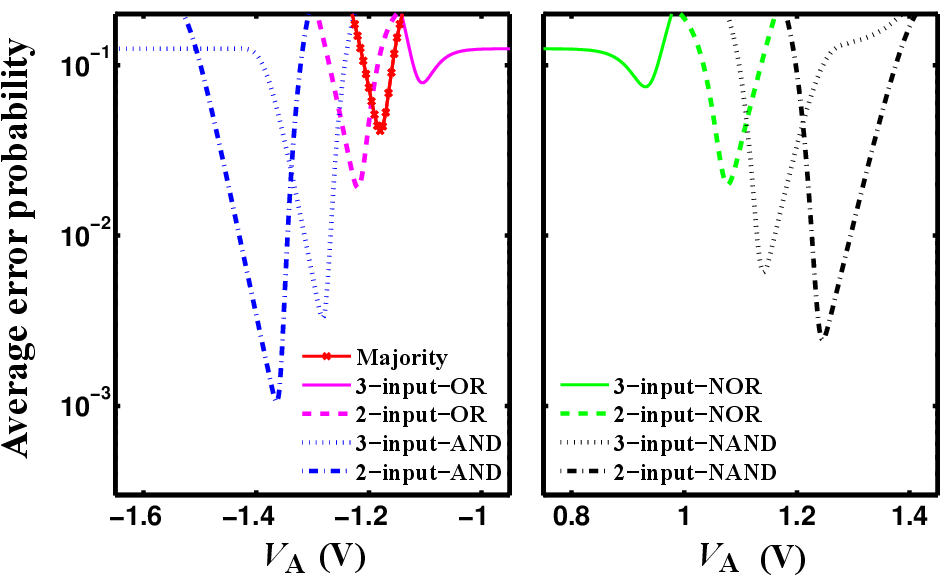Figure 4.20.: Average error probabilities for the basic reprogrammable operations as a function of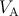.

Fig. 4.19 shows the different values of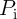for the AND (left) and the NAND (right) logic operations based on the two-input reprogrammable gate as a function of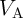. MTJs are characterized as,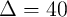,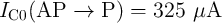, and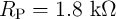. For a voltage level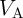chosen within the reliable gap (RG) opened between the switching windows (SWs) of the nearest desired (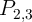) and undesired (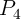) switching events, the average error probability is minimized. Fig. 4.20 shows the average error probabilities (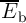) for different logic operations for the two- and three-input reprogrammable gates as a function of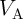. It illustrates that for each operation there is an optimal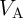and for both two- and three-input gates the operations AND and NAND offer higher reliability as compared to the other logic operations.

In fact, as it is shown in Table 4.1 and Table 4.2, the operations AND and NAND exhibit undesired switching when the inputs (and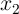) are in high-resistance state (State 4), but a desired switching when one of the inputs is in the low-resistance state (State 2 or State 3); and the operations OR and NOR exhibit a desired switching when the inputs (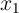and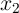) are in the low-resistance state (State 1) but undesired switching when one of the inputs is in the high-resistance state (State 2 or State 3). Eq.4.25 shows that the change in resistance at the input (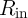) is higher when there is a modulation between State 4 and State 2 (or State 3) rather than a modulation between State 1 and State 2 (or State 3) (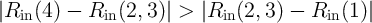).

 |ΔRin(4, 3)|-|ΔRin(3, 1)| =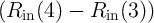- (Rin(3) - Rin(1)) =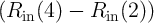- (Rin(2) - Rin(1)) =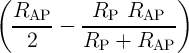-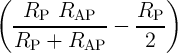=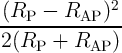> 0 (4.25)
It is clear that a higher resistance modulation in the input MTJs provides a higher current modulation in the output MTJ of a reprogrammable logic gate (Fig. 4.18). As a result, according to Eq.4.25, the current flowing through the output MTJ has a higher modulation, when State 2 (or State 3) is compared to State 4 rather than to State 1.
 |Rin(4) - Rin(2, 3)| > |Rin(2, 3) - Rin(1)| ⇒ |Iout(4) - Iout(2, 3)| > |Iout(2, 3) - Iout(1)| (4.26)
Therefore, the modulation between the nearest desired (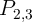) and undesired (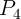) switching events for the (N)AND operation is higher than that of between the nearest desired (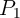) and undesired (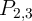) events for the (N)OR operation. Thus, the (N)AND operation provide a more reliable behavior (Fig. 4.20).

The logic implementation using MTJ-based logic gates relies on a state dependent current modulations on the output (target) MTJs. These modulations are caused by the changes in the MTJs’ resistances for different initial logic states. According to Eq. 4.18, the resistance modulation between the high and low resistance states in the MTJ with antiparallel and parallel magnetization alignments is proportional to the TMR ratio of the MTJs. Therefore, from a device point of view, the average error probabilities of all MTJ-based operations are expected to decrease with increasing TMR ratio. In fact, as we will see later, the TMR ratio is considered as a very important device parameter for the reliability of all STT-MTJ-based logic gates. The width of the reliable gap between the switching probabilities in the reprogrammable gate (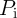in Fig. 4.19) is enlarged for a higher TMR ratio as the difference between the different input states originates from the modulation between the HRS and the LRS of the MTJs (Table 4.1 and Table 4.2). It is also clear that a resistance modulation (the difference between the HRS and the LRS states) of one input MTJ causes a higher current modulation in the output MTJ, when it is only connected (in parallel) to one other input MTJ rather than two input MTJs. Therefore, compared to the three-input reprogrammable gate, the two-input reprogrammable gate provides a higher current modulation in its output MTJ and thus exhibits a more reliable logic behavior (see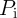for different operations in Fig. 4.20). Accordingly, in the following we employ only the two-input gate for the performance analysis and comparison between the reprogrammable and the implication logic architectures.